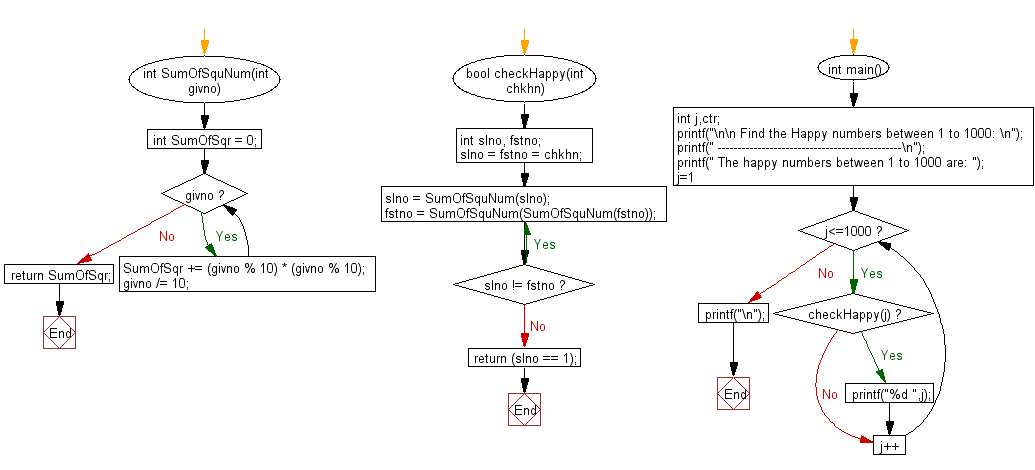﻿ C Program: Find the happy numbers between 1 to 1000 - w3resource# C Exercises: Find the happy numbers between 1 to 1000

## C Numbers: Exercise-14 with Solution

Write a program in C to find the happy numbers between 1 to 1000.

Sample Solution:

C Code:

``````# include <stdio.h>
# include <math.h>
# include <stdlib.h>
# include <stdbool.h>

int SumOfSquNum(int givno)
{
int SumOfSqr = 0;
while (givno)
{
SumOfSqr += (givno % 10) * (givno % 10);
givno /= 10;
}
return SumOfSqr;
}
bool checkHappy(int chkhn)
{
int slno, fstno;
slno = fstno = chkhn;
do
{
slno = SumOfSquNum(slno);
fstno = SumOfSquNum(SumOfSquNum(fstno));
}
while (slno != fstno);
return (slno == 1);
}
int main()
{
int j,ctr;
printf("\n\n Find the Happy numbers between 1 to 1000: \n");
printf(" ----------------------------------------------\n");
printf(" The happy numbers between 1 to 1000 are: ");

for (j=1;j<=1000;j++)
{
if (checkHappy(j))
printf("%d ",j);

}
printf("\n");
}
```
```

Sample Output:

``` The happy numbers between 1 to 1000 are: 1 7 10 13 19 23 28 31 32 44 49.....
```

Pictorial Presentation:Flowchart:C Programming Code Editor:

What is the difficulty level of this exercise?

Test your Programming skills with w3resource's quiz.

﻿

## C Programming: Tips of the Day

Why do C and C++ compilers allow array lengths in function signatures when they're never enforced?

It is a quirk of the syntax for passing arrays to functions.

Actually it is not possible to pass an array in C. If you write syntax that looks like it should pass the array, what actually happens is that a pointer to the first element of the array is passed instead.

Since the pointer does not include any length information, the contents of your [] in the function formal parameter list are actually ignored.

Ref : https://bit.ly/3fhlvdH